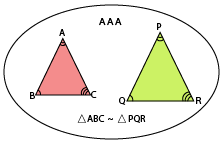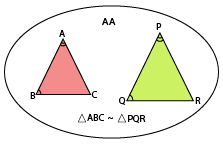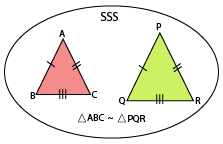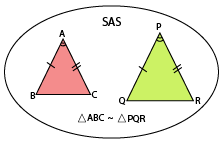Similarity of two triangles

## Objective

To establish the criteria for similarity of two triangles

## Prerequisite Knowledge

• Concept of congruence

• One-to-one correspondence

• Basic proportionality theorem

• Angle and Side measurement skills

## Theory

Two triangles are similar if:

• Their corresponding angles are equal.
• Their corresponding sides are in the same ratio or proportion.

Similar triangles are triangles that have the same shape, but their sizes may vary. All equilateral triangles and squares of any side length are examples of similar objects.

In other words, if two triangles are similar, then their corresponding angles are congruent and their corresponding sides are in equal proportion.

### Criteria for Similarity of the triangle

• AAA (Angle-Angle-Angle)

• AA (Angle-Angle)

• SSS (Side-Side-Side)

• SAS (Side-Angle-Side)

AAA (Angle-Angle-Angle)The Angle-Angle-Angle (AAA) criterion for the similarity of triangles states that “If in two triangles, corresponding angles are equal, then their corresponding sides are in the same ratio (or proportion) and hence the two triangles are similar”.

AA (Angle-Angle)The AA criterion states that "if two angles of a triangle are respectively equal to the two angles of another triangle, then the two triangles are similar".

This criterion is included in the AAA criteria itself.

SSS (Side-Side-Side)The Side-Side-Side (SSS) criterion for the similarity of two triangles states that “If in two triangles, sides of one triangle are proportional to (i.e., in the same ratio of ) the sides of the other triangle, then their corresponding angles are equal and hence the two triangles are similar”.

SAS (Side-Angle-Side)The Side-Angle-Side (SAS) criterion for the similarity of two triangles states that “If one angle of a triangle is equal to one angle of the other triangle and the sides including these angles are proportional, then the two triangles are similar”.